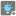• # Help with understanding two asserts

## Question related to missionMultiple Lightbulbs

We have two asserts as:

```assert sum_light([
datetime(2015, 1, 12, 10, 0, 0),
datetime(2015, 1, 12, 10, 0, 10),
(datetime(2015, 1, 12, 10, 0, 0), 2),
(datetime(2015, 1, 12, 10, 1, 0), 2),
]) == 60

assert sum_light([
datetime(2015, 1, 12, 10, 0, 0),
datetime(2015, 1, 12, 10, 0, 10),
(datetime(2015, 1, 12, 11, 0, 0), 2),
(datetime(2015, 1, 12, 11, 1, 0), 2),
]) == 70
```

For the first assert the zeroth element in the input array "els" is a datetime object. The mission statement describes this as

```..a datetime object(which means the time when the first button was pressed)
```

In this case I interpret this to be the time the first button was pressed which turns on light bulb number 2 as bulb number 2 is the only numbered bulb in all the tuples and bulb number 2 also a datetime which matches the datetime of the zeroth element. Correct ?

The element at index 1 is also a datetime object. What does this mean for this object ? Is it the button press for some unknown light bulb number? Unknown because there is no tuple object with a datetime object that coincides with it ?

Maybe another way to frame my question is can you explain how 60 is arrived at for the first assert and 70 for the second please?36